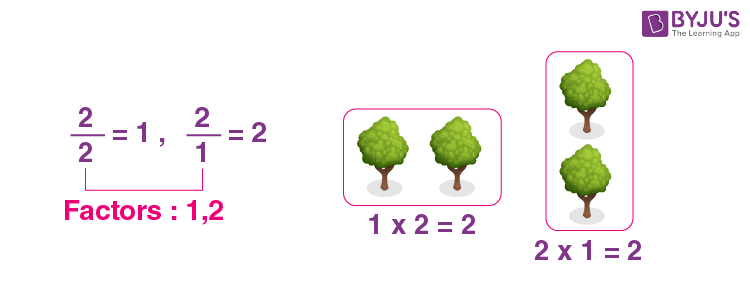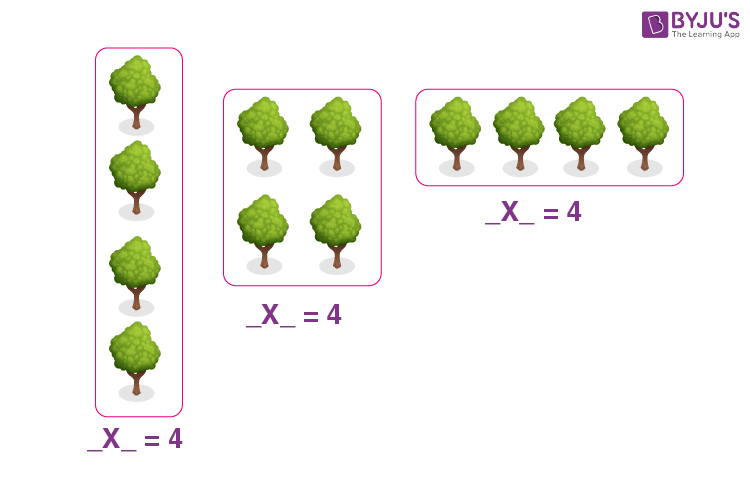Jet Set Go! All about Aeroplanes Jet Set Go! All about Aeroplanes

# Factors

In Mathematics, factors are the positive integers that can divide a number evenly.  Suppose we multiply two numbers to get a product. The number that is multiplied are the factors of the product. Each number is a factor of itself.  Factors have many real-life examples, such as arranging sweets in a box, arranging numbers in a pattern, distributing chocolates among children, etc. To find the factors of a number, we need to use the multiplication or division method.

## What are the Factors?

Factors are the numbers that can divide a number exactly.  Hence, after division, there is no remainder left. Factors are the numbers you multiply together to get another number. Thus, a factor is the divisor of another number.

## Examples of Factors

The process of finding the factors for a given number is better understood by making suitable arrangements. For example, to find the factors of 2, we have to arrange two objects differently. These arrangements are useful for writing the factors of a number. The below figure shows the factors of 2.Now, let’s find the factors of the smallest composite number, i.e. 4. Here, 4 objects are arranged in 3 different ways. Based on these arrangements, factor pairs and the factors of 4 are given.In the above figure, 4 objects are arranged in 3 ways, i.e.

• One column with 4 objects
• 2 rows and 2 columns (that means two rows/column with 2 objects each)
• One row with 4 objects

Thus, factor pairs are (1, 4), (2, 2) and (4, 1).

Factors are 1, 2, and 4.

Similarly, we can find the factors of 6. This can be observed in the following figure.In the above example, the factors of 6 are expressed in a different way. Arrangements are given for visualisation, whereas the division of numbers has been given to provide you with another way of getting the factors for a given number.

Therefore, the factors of 6 are 1, 2, 3, and 6.

### Factors of Prime and Composite Numbers

A prime number has only two factors, i.e., 1 and the number itself. But the composite numbers have more than two factors.

 Factors of Prime Numbers Factors of Composite Numbers 2 → 1, 2 4 → 1, 2, 4 5 → 1, 5 10 → 1, 2, 5, 10 17 → 1, 17 25 → 1, 5, 25 23 → 1, 23 32 → 1, 2, 4, 8, 16, 32

## Properties of Factors

Some of the important properties of factors of a number are listed below:

• 1 is a factor of every number
• Every natural number is a factor of itself
• Apart from 0 and 1, all the whole numbers have at least two factors
• Every factor is less than or equal to the given number
• The number of factors of a given number is finite
• Factors can be evaluated using both multiplication and division method

Now, one may get questions on how many factors a number has and how to find these factors. In this section, you will understand the process of finding factors of a given number clearly.

## How to Find Factors?

As we have already discussed, there are two possible methods to find factors of any number, such as:

• Multiplication
• Division

### Finding Factors using Multiplication

Since multiplication of two numbers results in a product such that the two numbers become the factors of the product. Thus, to find the factors, we need to follow the below steps:

• If we need to find the factors of a number, say N, then write the multiplication of two numbers in different ways, such that the resulting value is equal to N
• All the individual numbers that results in the product equal to N are the factors

Let us see an example: What are the factors of 36?

We can find the factors of 36 using multiplication as given below:

• 1 × 36 = 36
• 2 × 18 = 36
• 3 × 12 = 36
• 4 × 9 = 36
• 6 × 6 = 36

Now we have got the repeated numbers in the multiplication. Hence, we should stop writing 36 as the product of other numbers.

Thus, the factors of 36 are 1, 2, 3, 4, 6, 9, 12, 18, and 36.

### Finding Factors Using Division

Suppose a number N is given for which we need to find the factors. Now follow the below-given steps to find the factors of N using the division method:

• Figure the numbers that are less than N
• Now, divide N from the numbers such that a resulting quotient is a whole number
• Thus, all the divisors and quotients involved here become the factors of N, without any repetition

Example: Find factors of 6.

The numbers less than 6 are 1, 2, 3, 4, and 5.

Let us divide 6 by each of these numbers

• 6/1 = 6
• 6/2 = 3
• 6/ 3 = 2
• 6/4 = 1 (remainder = 2)
• 6/5 = 1 (remainder = 1)
• 6/6 = 1

Thus we can see, apart from 4 and 5, all the other numbers divide 6 evenly. Hence, 1, 2, 3 and 6 are the factors of 6.

## Solved Problems on Factors

Q.1: Find factors of 24.

Solution:

Let’s write the number 24 as the product of numbers.

• 1 × 24 = 24
• 2 × 12 = 24
• 3 × 8 = 24
• 4 × 6 = 24
• 6 × 4 = 24

Now, there is repeated multiplication, so we have to stop the multiplication.

Hence, the factors of 24 are: 1, 2, 3, 4, 6, 12 and 24

Q.2: What are the Factors of 72?

Solution: Representation 72 as the product of other numbers is given as:

• 1 × 72 = 72
• 2 × 36 = 72
• 3 × 24 = 72
• 4 × 18 = 72
• 6 × 12 = 72
• 8 × 9 = 72
• 9 × 8 = 72

Now, we have repeated multiplication so we have to stop the multiplication.

Thus, the factors of 72 are: 1, 2, 3, 4, 6, 8, 9, 12, 18, 24, 36 and 72

Q.3: Calculate the factors of 12.

Solution: Finding the factors of 12 is simple as given below:

• 1 × 12 = 12
• 2 × 6 = 12
• 3 × 4 = 12
• 4 × 3 = 12

Therefore, the factors of 12 are: 1, 2, 3, 4, 6 and 12

## Factors of Perfect Numbers

A number for which the sum of all its factors is equal to twice the number is called a perfect number. From this definition, we can say that the numbers 6 and 28 are perfect. This can be proved as given below:

As mentioned above, the factors of 6 are 1, 2, 3 and 6.

Now, we have to find the factors of 28.

1 × 28 = 28

2 × 14 = 28

4 × 7 = 28

7 × 4 = 28

Thus, the factors of 28 are 1, 2, 4, 7, 14 and 28.

Let’s add the factors of these numbers.

Sum of the factors of 6:

1 + 2 + 3 + 6 = 12 = 2 × 6 (that means twice the number)

Sum of the factors of 28:

1 + 2 + 4 + 7 + 14 + 28 = 56 = 2 × 28 (that means twice the number)

Therefore, 6 and 28 are perfect numbers.

The below table shows the list of factors of numbers. This will help you in solving the problems involving factors, common factors and prime factors in Maths.

## Factors in Algebra

Like natural numbers, factors are also defined for algebraic expressions. For example, the factors of 8x are 1, 2, 4, 8, x and 8x. Here, 8x is an algebraic expression, where 8 is the coefficient of variable x.

8x = 8 × x

There are factorisation methods to find the factors of such expressions. To learn more, click on the below links:

## List of Factors

Find the factors of more numbers in the below table.

## Frequently Asked Questions on Factors

### What are the factors of 12?

The factors of 12 are 1, 2, 3, 4, 6 and 12. These can be represented using multiplication as: 1 × 12 = 12, 2 × 6 = 12, 3 × 4 = 12.

### How do you explain factors?

Factors can be defined as the exact divisors of a given number. Also, factors are the numbers that are multiplied together (suitable combinations) to produce the original number.

### What are factors of 16?

The factors of 16 are 1, 2, 4, 8 and 16. The factors of 16 can be expressed in terms of multiplication of numbers as given below:
1 × 16 = 16
2 × 8 = 16
4 × 4 = 16

### What are the factors for 42?

The factors of 42 are: 1, 2, 3, 6, 7, 14, 21 and 42

### What are factors of 90?

The factors of 90 are: 1, 2, 3, 5, 6, 9, 10, 15, 18, 30, 45, and 90

### What are factors of 81?

The factors of 81 are: 1, 3, 9, 27 and 81